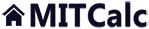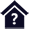#Conversion of units

Clicking on an item from the content will take you to the relevant paragraph.

## Conversion of units

The workbook contains a table for conversion of units, material hardness conversion and table of roughness achievable through different machining methods.

User interface.

Purchase, Price list.

## 1.0 Conversion of units.

Type the value you want to convert into the input box on the left. Select source units and target units on the right (there are two options).

## 2.0 Hardness table.

The table contains comparison values for different methods for measuring material hardness. The table also includes corresponding values of material hardness. A description (denomination) of the measuring method is specified in the note in the first line of the table.

## 3.0 Roughness table.

The table specifies the roughness values for different methods of machining:

• In green - achievable under standard conditions.
• In yellow - achievable under special conditions.

## 5.0 Oil viscosity.

In the graphs, the temperature curves of individual viscosity classes are displayed. On the line [5.1] you can switch between the degrees of Celsius and Fahrenheit. The line [5.2] is used to select curves for the viscosity index of 0,50,95.

If you need the exact viscosity for the specific temperature and the viscosity class, select the viscosity class on the line [5.4] and enter the temperature on the line [5.5]. There is a red cross shown in the graph, and the exact value for the selected curve and the specific temperature is mentioned on the line [5.6]. The line [5.7] shows the range for the selected viscosity class.

If you know the viscosity for two temperatures and you need to know the viscosity for a different temperature, enter the temperature and viscosity values on the lines [5.8-5.13]. The resulting viscosity is shown on the line [5.14].

The viscosity is calculated with use of the formulas in the standard (ASTMD341):

log(log(v + 0.7)) = A - B * log(T) ... [T...Kelvin, v...cSt, A,B ... constants]
B = {log(log(v2 + 0.7)) - log(log(v1+0.7))} / (log(T1)-log(T2))
A = log(log(v2 + 0.7)) + B * log(T2)
v = 10^(10^(A - B * log(T))) - 0.7

The curve that is displayed in red in the graph is then defined by two points (T1, T2, v1, v2) in the column marked as "User curve".

### 5.2 Viscosity index

The number characteristic for the viscosity dependence on the temperature. The higher the viscosity index, the lower the viscosity dependence on the temperature.